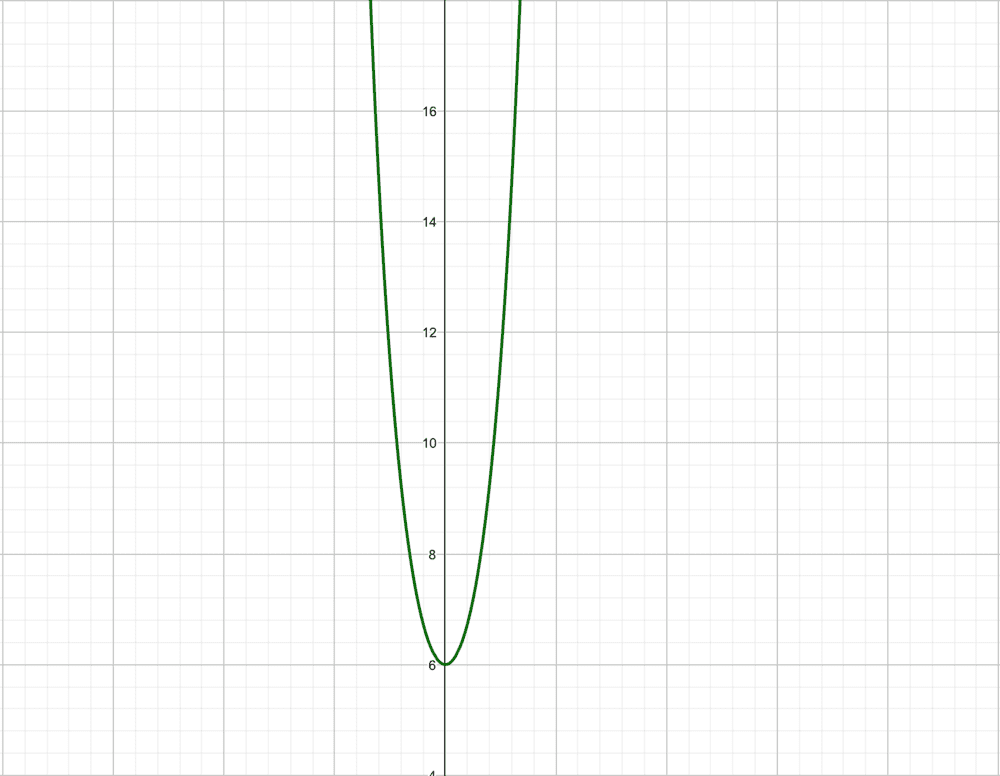# Finding the Range of the given function

Homework Statement:
Find the range of ##f(x) = 6^x + 3^x + 6^{-x} + 3^{-x} + 2##
Relevant Equations:
AM >= GM
##f(x) = 6^x + 3^x + 6^{-x} + 3^{-x} + 2##

But, ## AM >= GM##

So,

##f(x) >= 5 * 2 ^ {\frac{1}{5}}##

But this is not the case. According to the graph, it is ## f(x) >= 6##.If I do the same thing without considering the constant '2' then I am getting the answer.

let ##g(x) = 6^x + 3^x + 6^{-x} + 3^{-x} ## and ##f(x) = g(x) + 2##

Using ##AM>=GM##,

##g(x) >= 4##

Hence, ##f(x) >= 6##

Why is this the case? Why is the latter approach working but no the former?

#### Attachments

Mark44
Mentor
Homework Statement:: Find the range of ##f(x) = 6^x + 3^x + 6^{-x} + 3^{-x} + 2##
Relevant Equations:: AM >= GM

##f(x) = 6^x + 3^x + 6^{-x} + 3^{-x} + 2##
Kaushik said:
But, ## AM >= GM##
So,
##f(x) >= 5 * 2 ^ {\frac{1}{5}}##
I don't see what AM >= GM has to do with this problem, or how the inequality you show is related to this problem. Certainly ##f(x) \ge 5 \cdot 2^{1/5}##, but that's a very rough lower bound.
Kaushik said:
But this is not the case. According to the graph, it is ## f(x) >= 6##.

View attachment 258864

If I do the same thing without considering the constant '2' then I am getting the answer.

let ##g(x) = 6^x + 3^x + 6^{-x} + 3^{-x} ## and ##f(x) = g(x) + 2##

Using ##AM>=GM##,

##g(x) >= 4##

Hence, ##f(x) >= 6##

Why is this the case? Why is the latter approach working but no the former?
##6^x + 6^{-x}## has a minimum value of 2 for x = 0. Likewise, ##3^x + 3^{-x}## also has a minimum value of 2 at x = 0. Add these pairs together + 2, and you get a minimum value of 6.

•